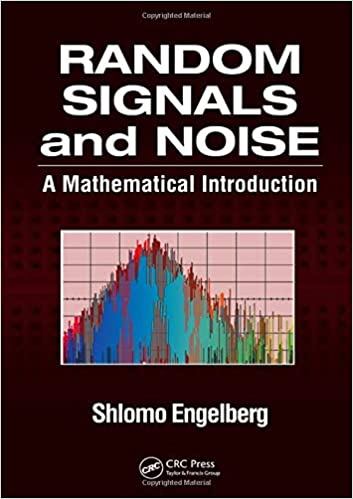# Random Signals and Noise: A Mathematical Introduction (Instructor Resources)English | 2006 | ISBN-13: 978-0849375545 | Instructor Resources | PDF/Solution Manual | 0.8 MB

Understanding the nature of random signals and noise is critically important for detecting signals and for reducing and minimizing the effects of noise in applications such as communications and control systems. Outlining a variety of techniques and explaining when and how to use them, Random Signals and Noise: A Mathematical Introduction focuses on applications and practical problem solving rather than probability theory.